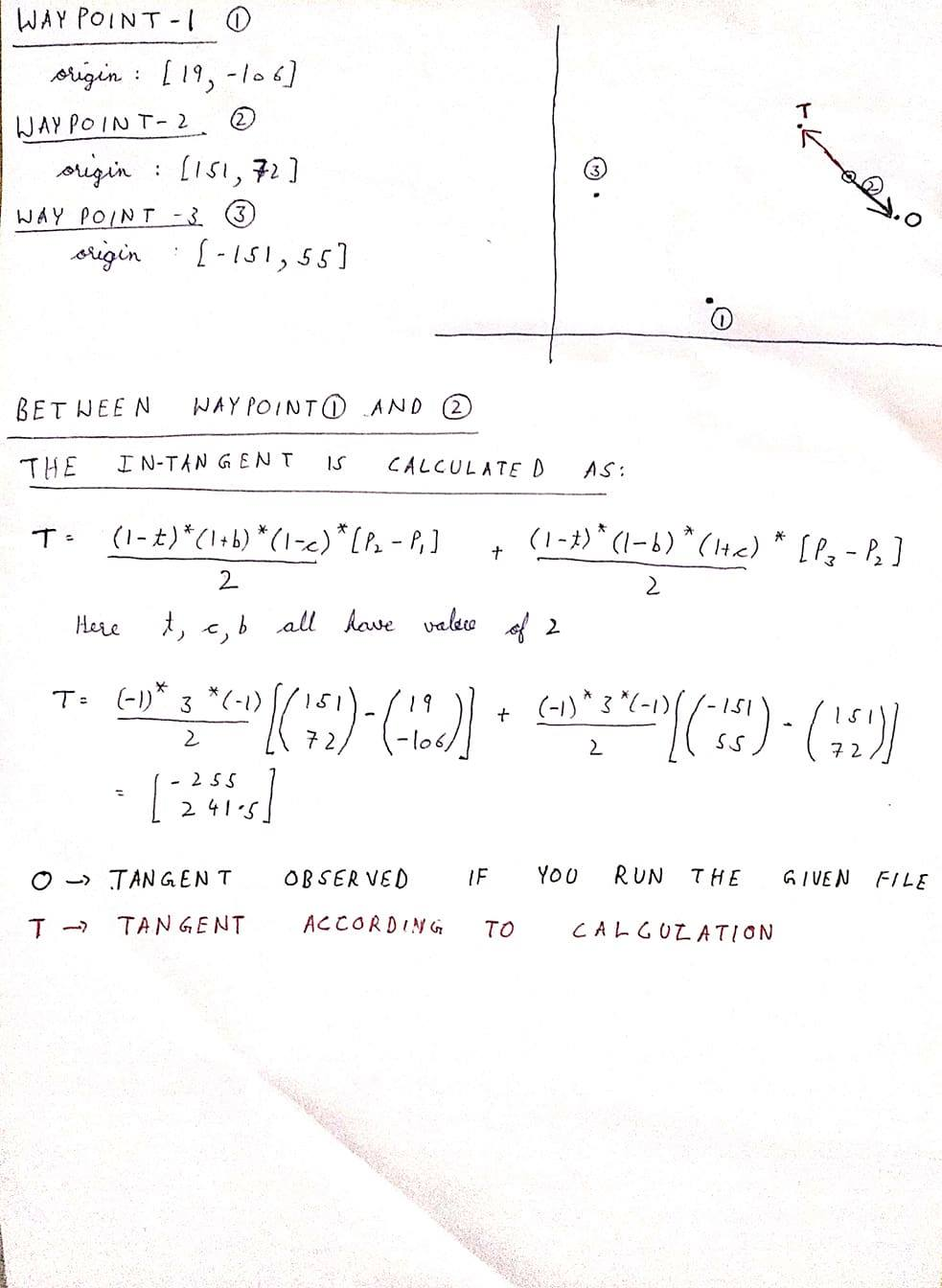# [Solved] Unexpected tangent(interpolation) behaviour between two keyframes

#1

Hello,
I was trying to study how the tangents are decided/calculated from the TCB values. So I came across this file and saw how the in-tangent and out-tangent are calculated. It uses bezier between two waypoints.
Now I created a star layer and three waypoints and tried to predict there motion using the in-tangents and out-tangents but got some unexpected results.
The file I am using is MovingStar.sifz (861 Bytes)

All the waypoints used have TCB interpolation methods(both in and out).
I will show the calculation of in-tangent at waypoint 2:I am assuming that all the tangents are calculated with respect to the waypoints. That is the in-tangent is calculated with respect to waypoint-2. Please correct me if I got this wrong.

Now in the above image, “`T`” represents what I expected to be the tangent should have been. But when you open the attached file in synfig and run it, you will get the tangent “`O`” approximately(I don’t know how to find the exact co-ordinates).
Please tell me where am I going wrong or did I miss something?

#2

#3

The equation that you have show is for out tangent from point P1.
The one I wrote is for the in tangent for point P2.
Sorry for the confusion, I guess pointed towards the wrong line in the mentioned link. The correct one is this.. I will edit this in the main topic also.
Thank you!

#4

It looks like the case you referenced ( this) doesn’t happens for P2.

The condition is:

``````/// iter          next          after_next
/// ANY/ANY ------TCB/ANY ----- ANY/ANY
///
if(next_get_before==INTERPOLATION_TCB && after_next!=animated.waypoint_list_.end() && !is_angle())
``````

As I understand, there are no “after next” point for P2 in your example, so `after_next!=animated.waypoint_list_.end()` is `False`.

#5

Please note that there are three points P1, P2 and P3.
So my `iter` is `P1`, `next` is `P2`, and `after_next` is `P3`, hence the code should go inside this “if” condition.
So we are calculating the out-tangent from `iter` and in-tangent for `next`.
Please correct me if I am wrong.

#6

So I got it where I was making a mistake. At waypoint-2, the in-tangent should look like `T` only. But I got confused because `lottie` format uses `O` as the in-tangent for waypoint-2.
So I guess this issue is resolved.
Thank you

#7

Ah, sorry. As you probably noticed, I do not have enough knowledge for this part of code yet.

Glad to hear you’ve got that sorted out!